# NCERT Solutions for Class 8 Math Chapter 12 - Exponents and Powers

##### Question 1:

Find the multiplicative inverse of the following:
(i) 2–4 (ii) 10–5 (iii) 7–2
(iv) 5–3 (v) 10 –100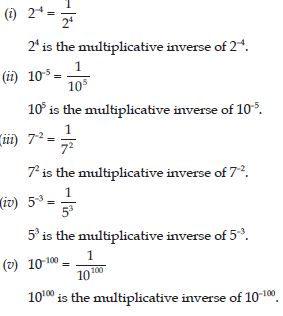##### Question 2:

Expand the following numbers using exponents:
(i) 1025.63 (ii) 1256.249.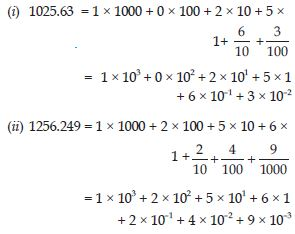##### Question 3:

Simplify and write in exponential form:
(i) (–2)–3 × (–2)–4 (ii) p3 × p–10
(iii) 32 × 3–5 × 36##### Question 4: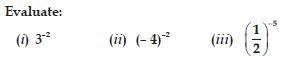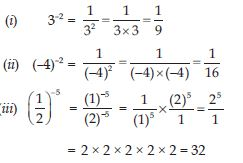##### Question 5: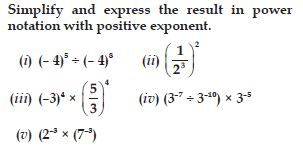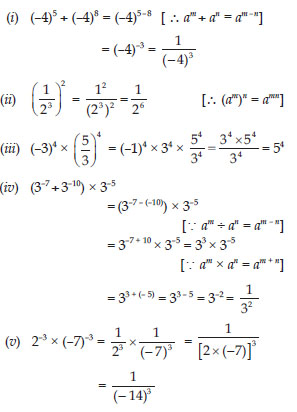##### Question 6: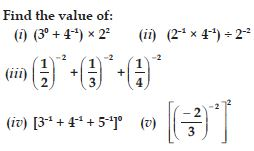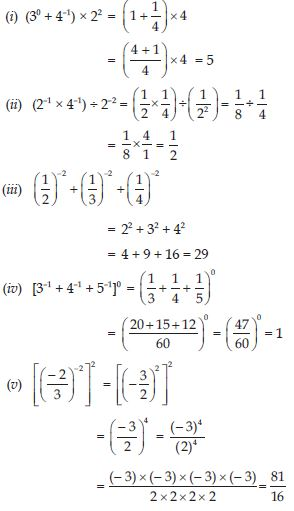##### Question 7: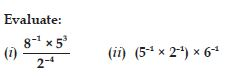##### Question 8: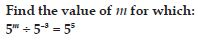##### Question 9: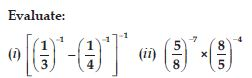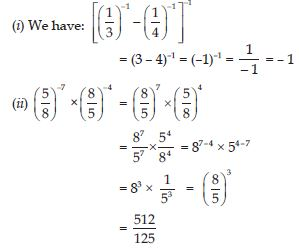##### Question 10: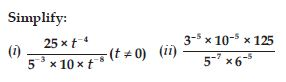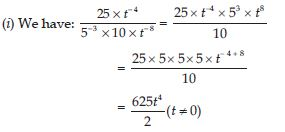##### Question 11:

Write the following numbers in standard form:
(i) 0.000000564 (ii) 0.0000021
(iii) 21600000 (iv) 15240000

(i) 0.000000564
Let decimal to be moved 7 places to the right.
$\therefore$ 0.000000564 = 5.64 × 10–7
(ii) 0.0000021
Let decimal to be moved 6 places to the right:
$\therefore$ 0.0000021= 2.1 × 10–6
(iii) 21600000
Let decimal to be moved 7 places to the left.
$\therefore$ 21600000 = 2.16 × 107
(iv) 15240000
Let decimal to be moved 7 places to the left.
$\therefore$ 15240000 = 1.524 × 107

##### Question 12:

Express the following numbers in standard form:
(i) 0.0000000000085 (ii) 0.00000000000942
(iii) 6020000000000000 (iv) 0.00000000837
(v) 31860000000

(i) 0.0000000000085
Let the decimal to be moved 12 places to the right.
$\therefore$ 0.0000000000085 = 8.5 × 10–12
(ii) 0.00000000000942
Let the decimal to be moved 12 places to the right.
$\therefore$ 0.00000000000942 = 9.42 × 10–12
(iii) 6020000000000000
Let the decimal to be moved 15 places to the left.
$\therefore$ 6020000000000000 = 6.02 × 1015
(iv) 0.00000000837
Let the decimal to be moved 9 places to the right.
$\therefore$ 0.00000000837 = 8.37 × 10–9
(v) 31860000000
Let the decimal to be moved 10 places to the left.
$\therefore$31860000000 = 3.186 × 1010

##### Question 13:

Express the following numbers in usual form:
(i) 3.02 × 10–6 (ii) 4.5 × 104
(iii) 3 × 10–8 (iv) 1.0001 × 109
(v) 5.8 × 1012 (vi) 3.61492 × 106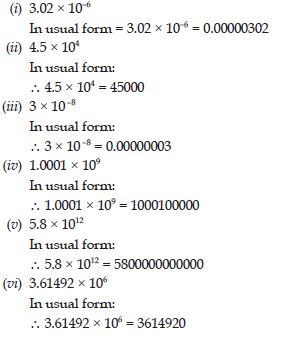##### Question 14:

Express the number appearing in the following statements in standard form: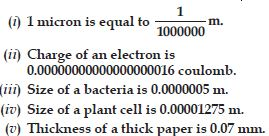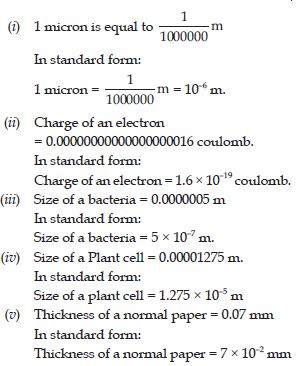##### Question 15:

In a stack there are 5 books each of thickness 20 mm and 5 paper sheets each of thickness 0.016 mm. What is the total thickness of the stack ?

$\therefore$ Thickness of 5 books = 20 × 5 = 100 mm
$\therefore$ Total thickness of stack